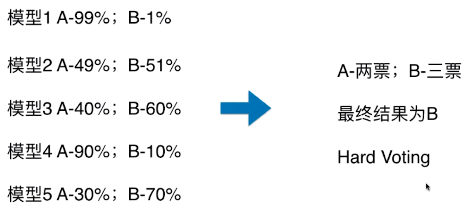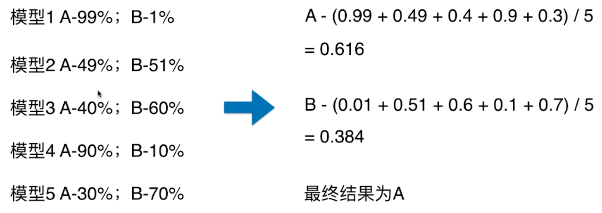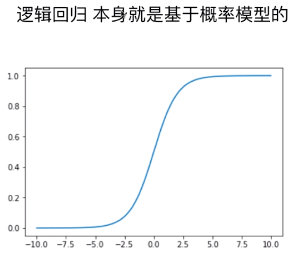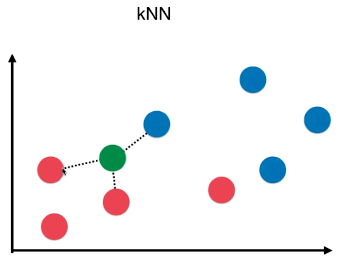# 【机器学习】集成学习（Soft Voting Classifier）

### 一、Hard Voting 与 Soft Voting 的对比

1）使用方式

• voting = 'hard'：表示最终决策方式为 Hard Voting Classifier；
• voting = 'soft'：表示最终决策方式为 Soft Voting Classifier；

2）思想

• Hard Voting Classifier：根据少数服从多数来定最终结果；
• Soft Voting Classifier：将所有模型预测样本为某一类别的概率的平均值作为标准，概率最高的对应的类型为最终的预测结果；
• ### Hard Voting

• 模型 1：A - 99%、B - 1%，表示模型 1 认为该样本是 A 类型的概率为 99%，为 B 类型的概率为 1%；
•• ### Soft Voting

• 将所有模型预测样本为某一类别的概率的平均值作为标准；
•• Hard Voting 投票方式的弊端
1. 如上图，最终的分类结果不是由概率值更大的模型 1 和模型 4 决定，而是由概率值相对较低的模型 2/3/5 来决定的；

### 二、各分类算法的概率计算

• Soft Voting 的决策方式，要求集合的每一个模型都能估计概率；

1）逻辑回归算法

• P = σ( y_predict )
•2）kNN 算法

• k 个样本点中，数量最多的样本所对应的类别作为最终的预测结果；
• kNN 算法也可以考虑权值，根据选中的 k 个点距离待预测点的距离不同，k 个点的权值也不同；
• P = n / k
• n：k 个样本中，最终确定的类型的个数；如下图，最终判断为 红色类型，概率：p = n/k = 2 / 3；
•3）决策树算法

• 通常在“叶子”节点处的信息熵或者基尼系数不为 0，数据集中包含多种类别的数据，以数量最多的样本对应的类别作为最终的预测结果；（和 kNN 算法类似）
• P = n / N
1. n：“叶子”中数量最多的样本的类型对应的样本数量；
2. N：“叶子”中样本总量；

4）SVM 算法

• 在 scikit-learn 中的 SVC() 中的一个参数：probability
1. probability = True：SVC() 返回样本为各个类别的概率；（默认为 False）
from sklearn.svm import SVC
svc = SVC(probability=True)

### 三、scikit-learn 中使用集成分类器：VotingClassifier

1）模拟数据集

import numpy as np
import matplotlib.pyplot as plt
from sklearn import datasets
from sklearn.model_selection import train_test_split

X, y = datasets.make_moons(n_samples=500, noise=0.3, random_state=42)
X_train, X_test, y_train, y_test = train_test_split(X, y, random_state=42)

2）voting = 'hard'：使用 Hard Voting 做决策

from sklearn.linear_model import LogisticRegression
from sklearn.svm import SVC
from sklearn.tree import DecisionTreeClassifier
from sklearn.ensemble import VotingClassifier

# 实例化
voting_clf = VotingClassifier(estimators=[
('log_clf', LogisticRegression()),
('svm_clf', SVC()),
('dt_clf', DecisionTreeClassifier(random_state=666))
], voting='hard')

voting_clf.fit(X_train, y_train)
voting_clf.score(X_test, y_test)
# 准确率：0.896

3）voting = 'soft'：使用 Soft Voting 做决策

voting_clf = VotingClassifier(estimators=[
('log_clf', LogisticRegression()),
('svm_clf', SVC(probability=True)),
('dt_clf', DecisionTreeClassifier(random_state=666))
], voting='soft')

voting_clf.fit(X_train, y_train)
voting_clf.score(X_test, y_test)
# 准确率：0.912
• 使用 Soft Voting 时，SVC() 算法的参数：probability=True

©️2019 CSDN 皮肤主题: 编程工作室 设计师: CSDN官方博客